Скачать презентацию 6 -1 Solving Systems by Graphing Preview Warm

39f931641b7a2ee867b6cb7cfa05a566.ppt

• Количество слайдов: 30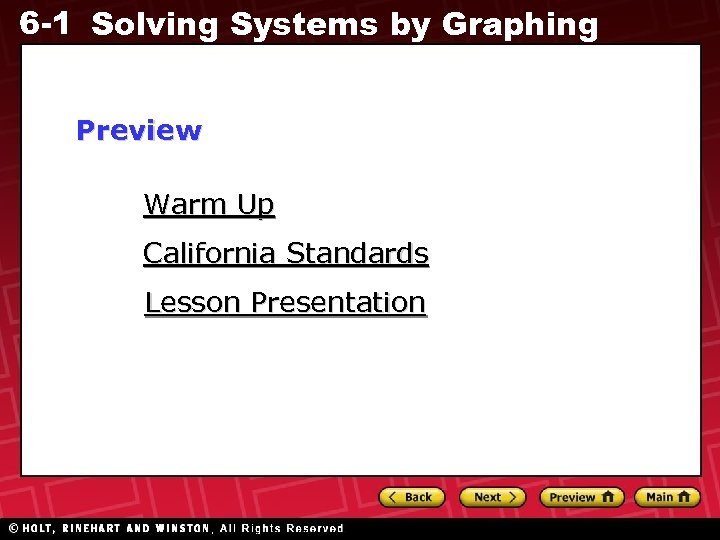6 -1 Solving Systems by Graphing Preview Warm Up California Standards Lesson Presentation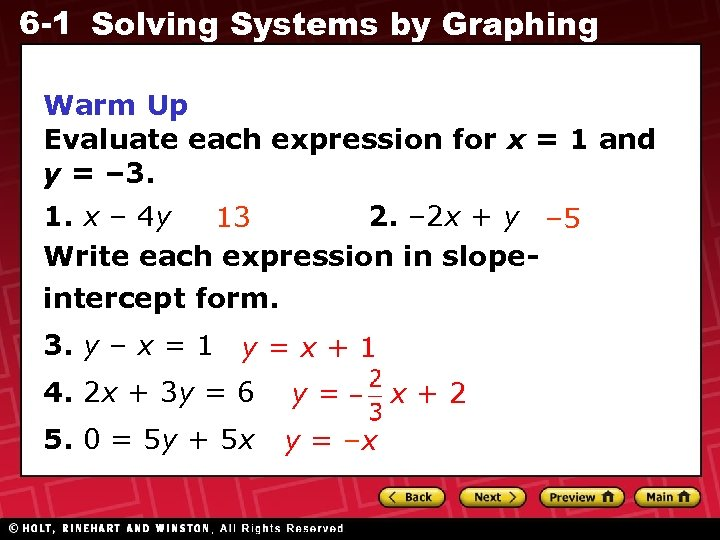6 -1 Solving Systems by Graphing Warm Up Evaluate each expression for x = 1 and y = – 3. 1. x – 4 y 2. – 2 x + y – 5 13 Write each expression in slopeintercept form. 3. y – x = 1 y = x + 1 4. 2 x + 3 y = 6 y= 5. 0 = 5 y + 5 x y = –x x+2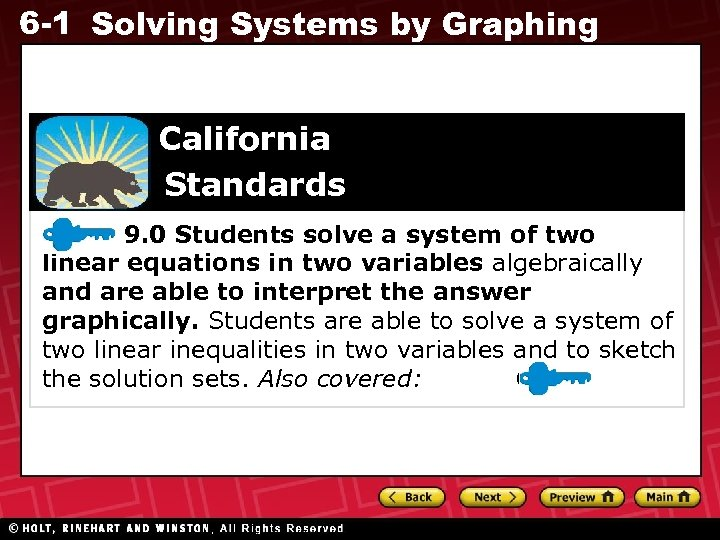6 -1 Solving Systems by Graphing California Standards 9. 0 Students solve a system of two linear equations in two variables algebraically and are able to interpret the answer graphically. Students are able to solve a system of two linear inequalities in two variables and to sketch the solution sets. Also covered: 6. 0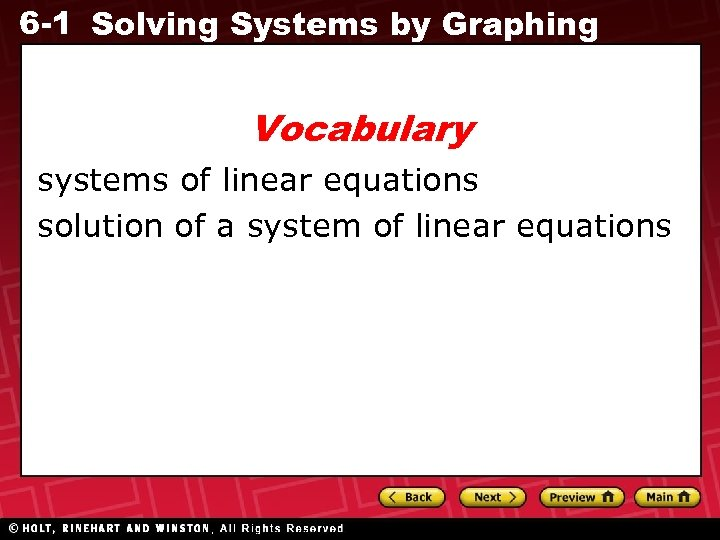6 -1 Solving Systems by Graphing Vocabulary systems of linear equations solution of a system of linear equations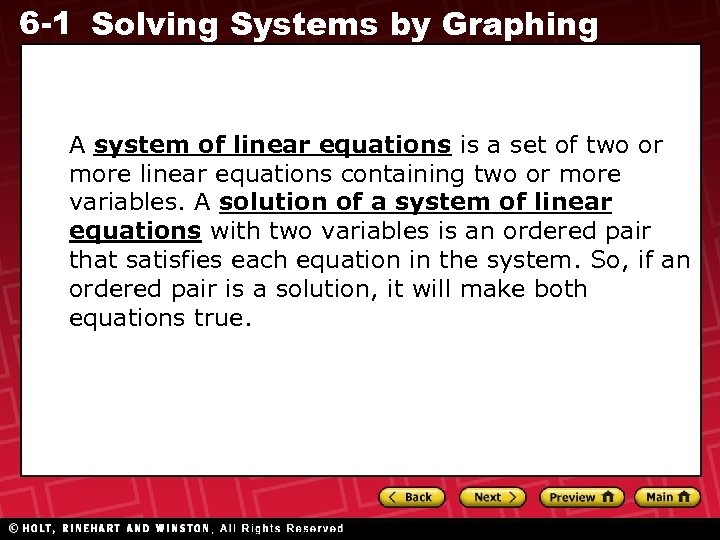6 -1 Solving Systems by Graphing A system of linear equations is a set of two or more linear equations containing two or more variables. A solution of a system of linear equations with two variables is an ordered pair that satisfies each equation in the system. So, if an ordered pair is a solution, it will make both equations true.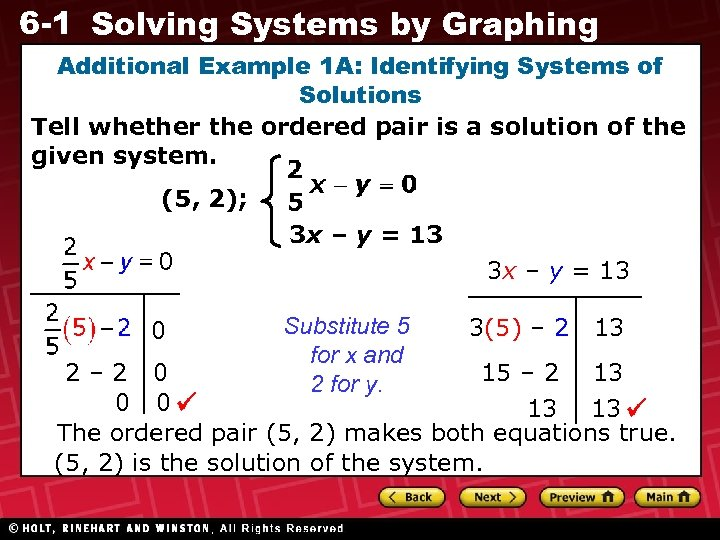6 -1 Solving Systems by Graphing Additional Example 1 A: Identifying Systems of Solutions Tell whether the ordered pair is a solution of the given system. (5, 2); 3 x – y = 13 0 Substitute 5 for x and 2 for y. 3(5) – 2 13 2– 2 0 15 – 2 13 0 0 13 13 The ordered pair (5, 2) makes both equations true. (5, 2) is the solution of the system.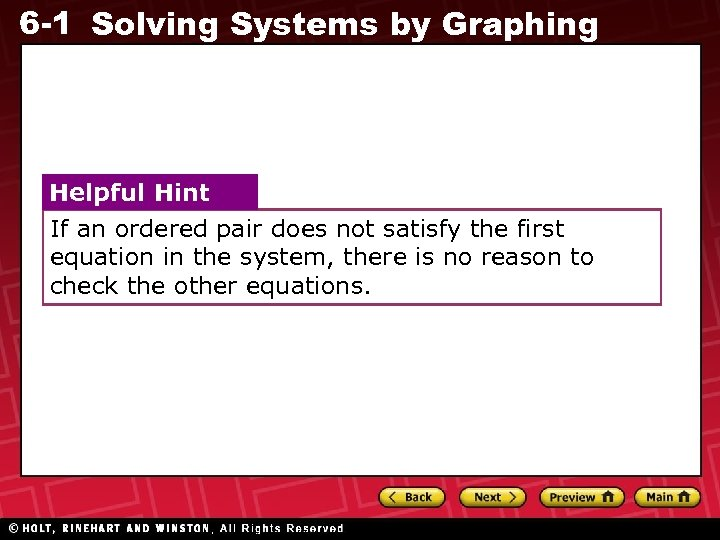6 -1 Solving Systems by Graphing Helpful Hint If an ordered pair does not satisfy the first equation in the system, there is no reason to check the other equations.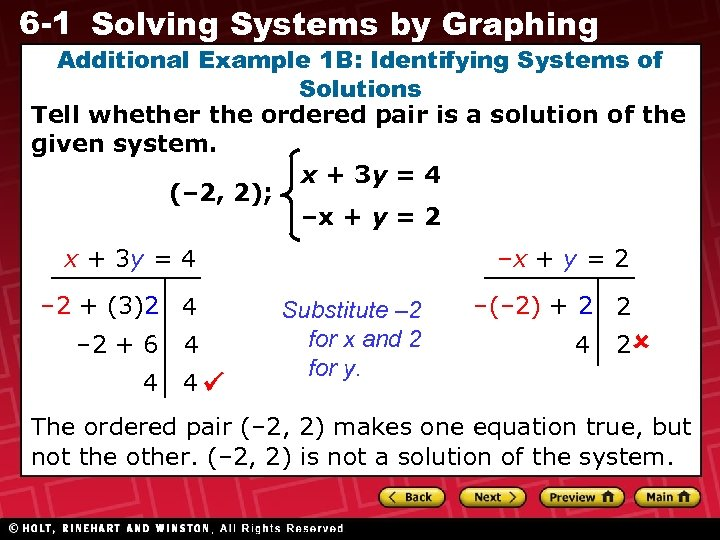6 -1 Solving Systems by Graphing Additional Example 1 B: Identifying Systems of Solutions Tell whether the ordered pair is a solution of the given system. x + 3 y = 4 (– 2, 2); –x + y = 2 x + 3 y = 4 – 2 + (3)2 4 – 2 + 6 4 4 4 –x + y = 2 Substitute – 2 for x and 2 for y. –(– 2) + 2 2 4 2 The ordered pair (– 2, 2) makes one equation true, but not the other. (– 2, 2) is not a solution of the system.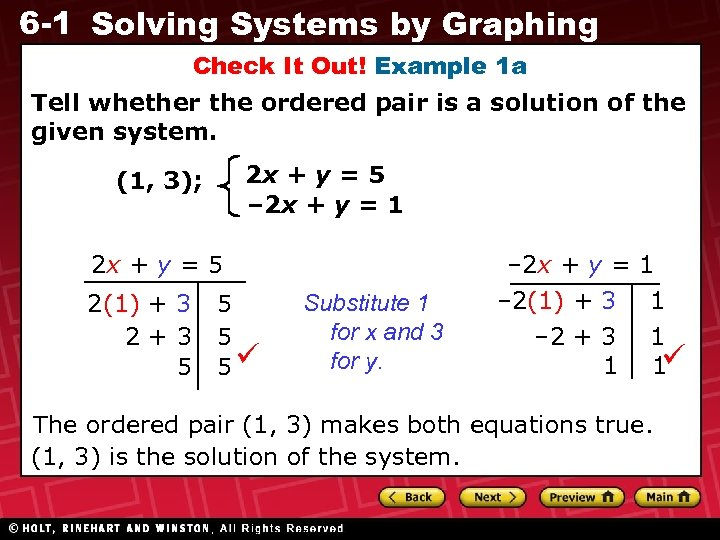6 -1 Solving Systems by Graphing Check It Out! Example 1 a Tell whether the ordered pair is a solution of the given system. 2 x + y = 5 – 2 x + y = 1 (1, 3); 2 x + y = 5 2(1) + 3 2+3 5 5 – 2 x + y = 1 Substitute 1 for x and 3 for y. – 2(1) + 3 – 2 + 3 1 1 1 1 The ordered pair (1, 3) makes both equations true. (1, 3) is the solution of the system.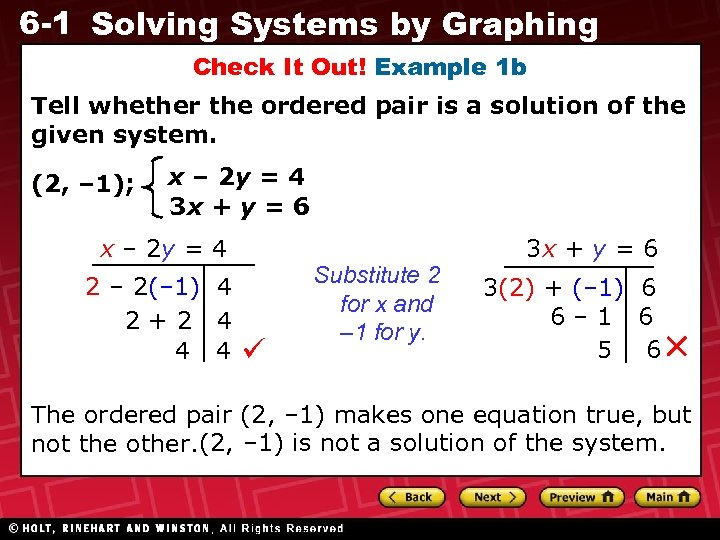6 -1 Solving Systems by Graphing Check It Out! Example 1 b Tell whether the ordered pair is a solution of the given system. (2, – 1); x – 2 y = 4 3 x + y = 6 x – 2 y = 4 2 – 2(– 1) 4 2+2 4 4 4 Substitute 2 for x and – 1 for y. 3 x + y = 6 3(2) + (– 1) 6 6– 1 6 5 6 The ordered pair (2, – 1) makes one equation true, but not the other. (2, – 1) is not a solution of the system.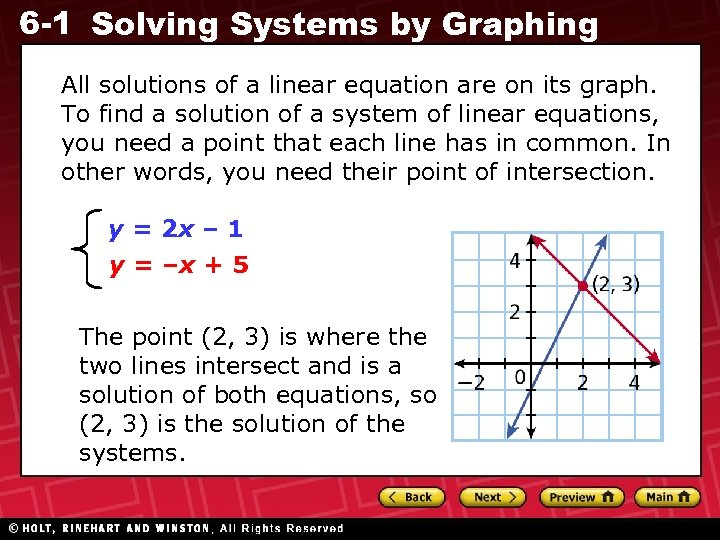6 -1 Solving Systems by Graphing All solutions of a linear equation are on its graph. To find a solution of a system of linear equations, you need a point that each line has in common. In other words, you need their point of intersection. y = 2 x – 1 y = –x + 5 The point (2, 3) is where the two lines intersect and is a solution of both equations, so (2, 3) is the solution of the systems.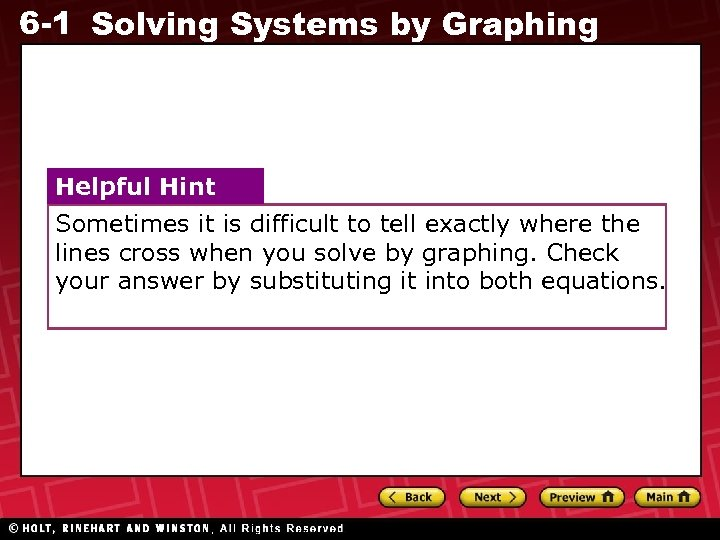6 -1 Solving Systems by Graphing Helpful Hint Sometimes it is difficult to tell exactly where the lines cross when you solve by graphing. Check your answer by substituting it into both equations.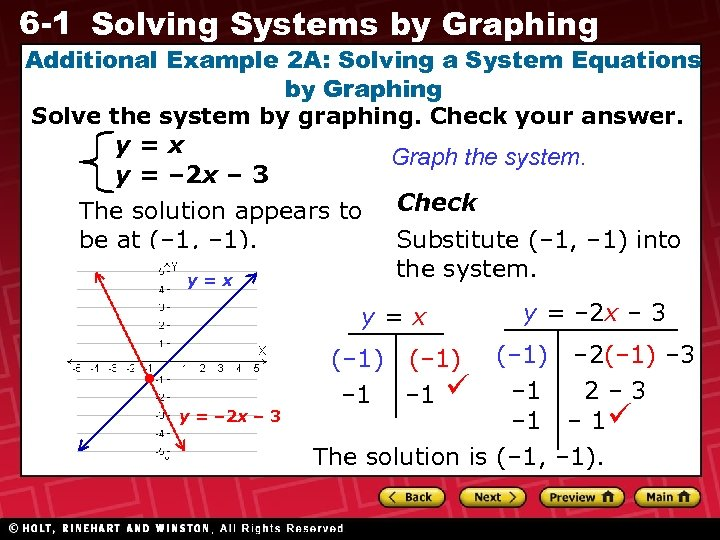6 -1 Solving Systems by Graphing Additional Example 2 A: Solving a System Equations by Graphing Solve the system by graphing. Check your answer. y=x Graph the system. y = – 2 x – 3 The solution appears to Check be at (– 1, – 1). Substitute (– 1, – 1) into the system. y=x • y = – 2 x – 3 (– 1) – 2(– 1) – 3 – 1 2– 3 – 1 The solution is (– 1, – 1). (– 1) – 1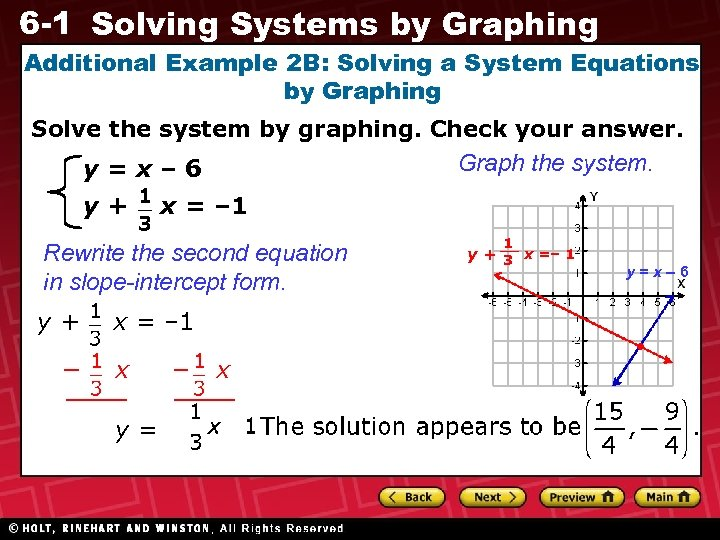6 -1 Solving Systems by Graphing Additional Example 2 B: Solving a System Equations by Graphing Solve the system by graphing. Check your answer. Graph the system. y=x– 6 y+ x = – 1 Rewrite the second equation in slope-intercept form. y+ − x = – 1 x y= − x 1 y + 3 x =– 1 y=x– 6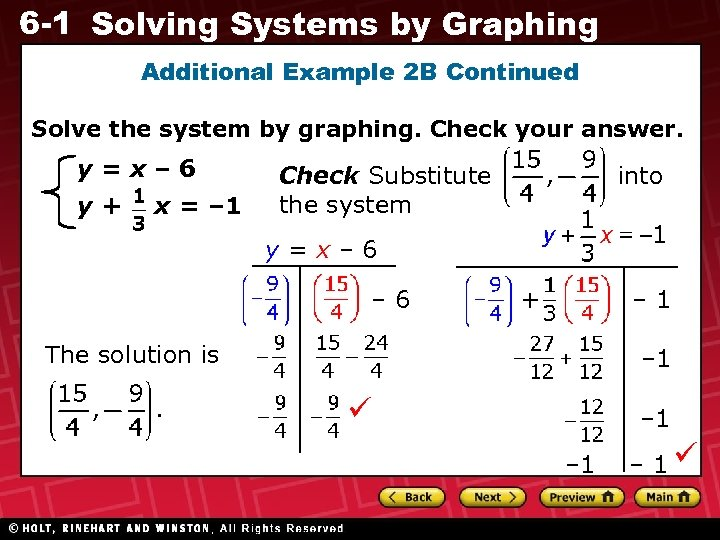6 -1 Solving Systems by Graphing Additional Example 2 B Continued Solve the system by graphing. Check your answer. y=x– 6 y+ x = – 1 Check Substitute the system into y=x– 6 + – 1 The solution is – 1 – 1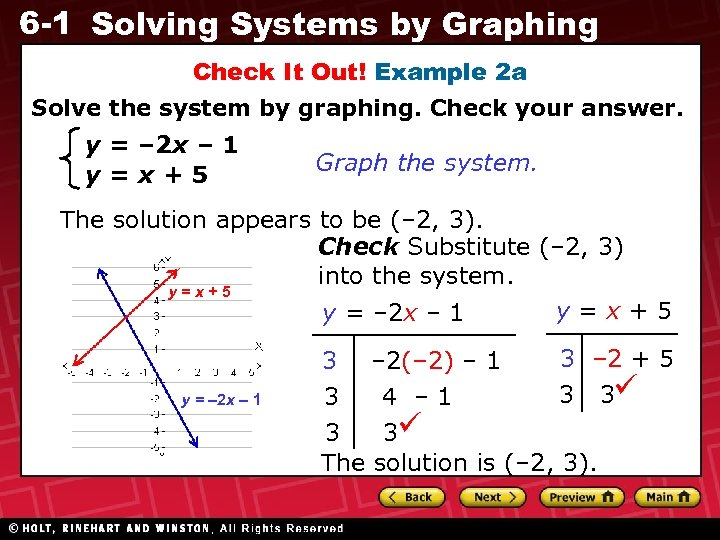6 -1 Solving Systems by Graphing Check It Out! Example 2 a Solve the system by graphing. Check your answer. y = – 2 x – 1 y=x+5 Graph the system. The solution appears to be (– 2, 3). Check Substitute (– 2, 3) into the system. y=x+5 y = – 2 x – 1 3 3 – 2(– 2) – 1 4 – 1 3 – 2 + 5 3 3 The solution is (– 2, 3).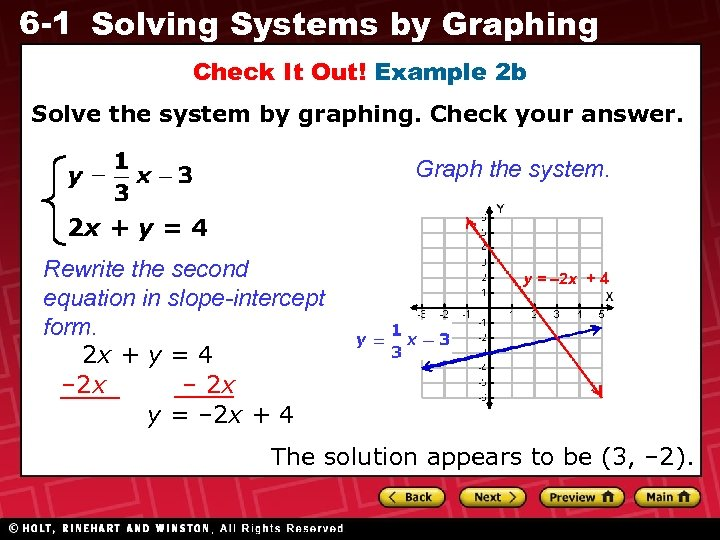6 -1 Solving Systems by Graphing Check It Out! Example 2 b Solve the system by graphing. Check your answer. Graph the system. 2 x + y = 4 Rewrite the second equation in slope-intercept form. 2 x + y = 4 – 2 x y = – 2 x + 4 The solution appears to be (3, – 2).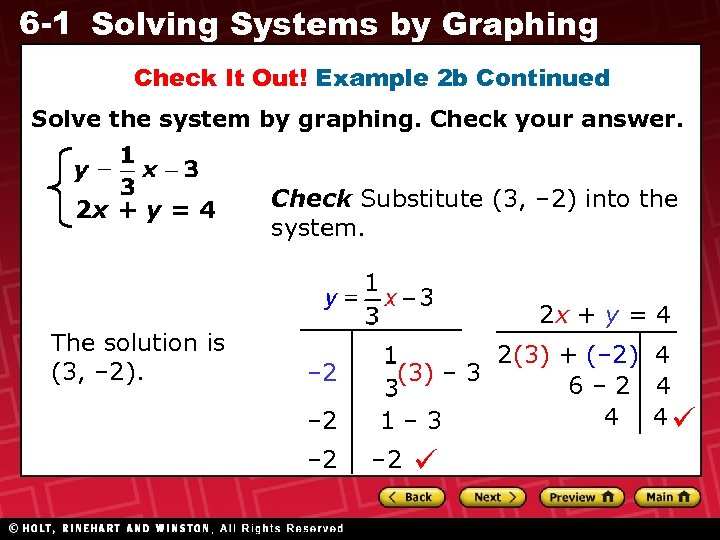6 -1 Solving Systems by Graphing Check It Out! Example 2 b Continued Solve the system by graphing. Check your answer. 2 x + y = 4 The solution is (3, – 2). Check Substitute (3, – 2) into the system. 2 x + y = 4 – 2 – 2 2(3) + (– 2) 4 (3) – 3 6– 2 4 4 4 1– 3 – 2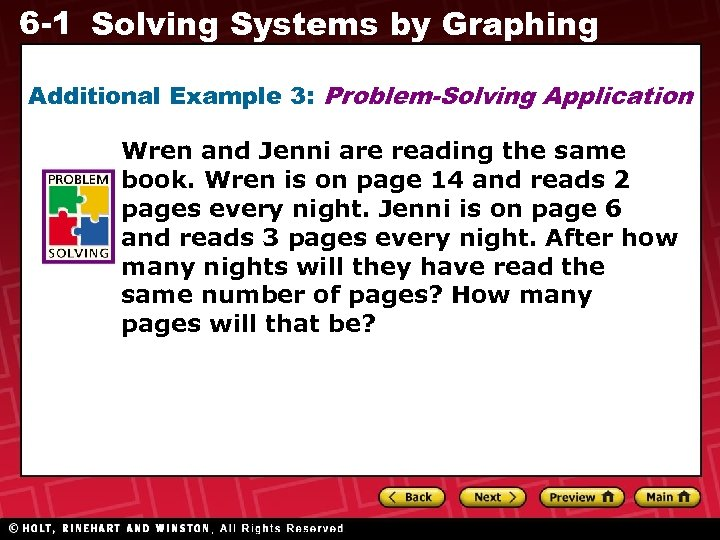6 -1 Solving Systems by Graphing Additional Example 3: Problem-Solving Application Wren and Jenni are reading the same book. Wren is on page 14 and reads 2 pages every night. Jenni is on page 6 and reads 3 pages every night. After how many nights will they have read the same number of pages? How many pages will that be?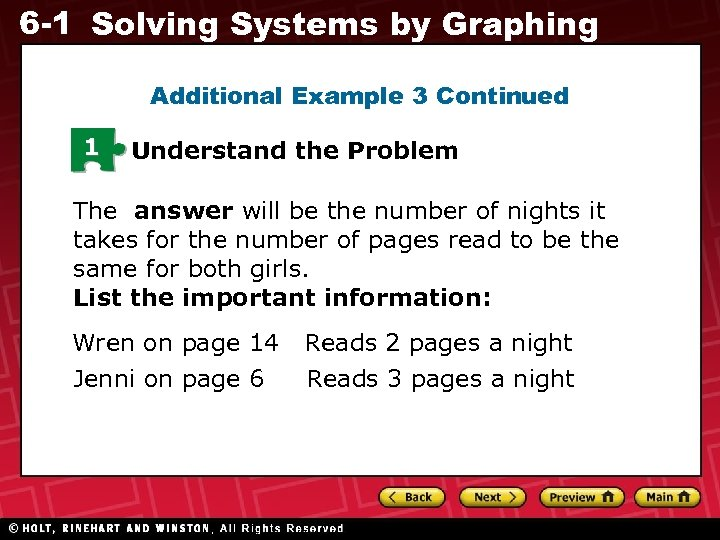6 -1 Solving Systems by Graphing Additional Example 3 Continued 1 Understand the Problem The answer will be the number of nights it takes for the number of pages read to be the same for both girls. List the important information: Wren on page 14 Jenni on page 6 Reads 2 pages a night Reads 3 pages a night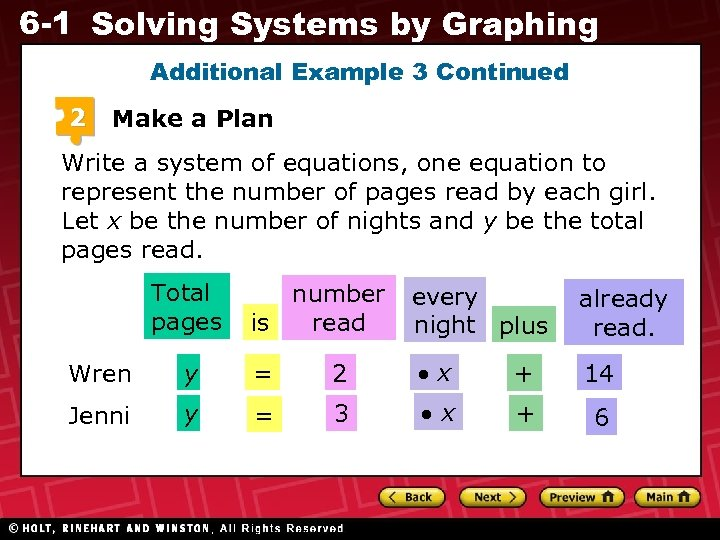6 -1 Solving Systems by Graphing Additional Example 3 Continued 2 Make a Plan Write a system of equations, one equation to represent the number of pages read by each girl. Let x be the number of nights and y be the total pages read. Total pages number read is every night plus already read. Wren y = 2 x + 14 Jenni y = 3 x + 6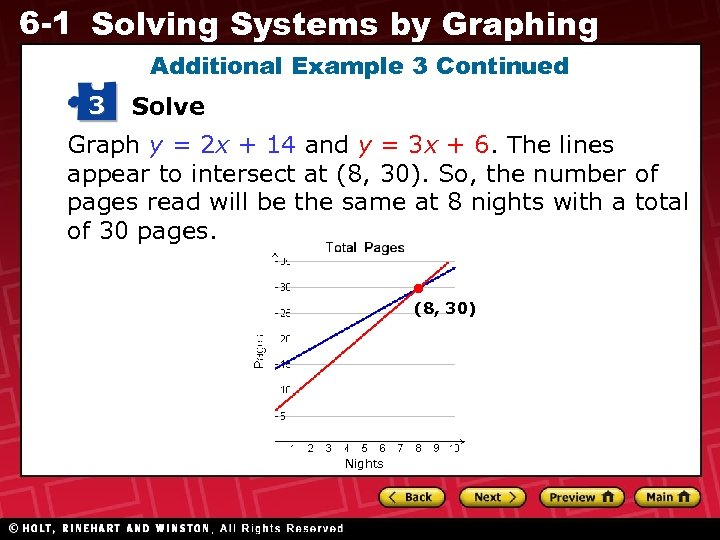6 -1 Solving Systems by Graphing Additional Example 3 Continued 3 Solve Graph y = 2 x + 14 and y = 3 x + 6. The lines appear to intersect at (8, 30). So, the number of pages read will be the same at 8 nights with a total of 30 pages. (8, 30) Nights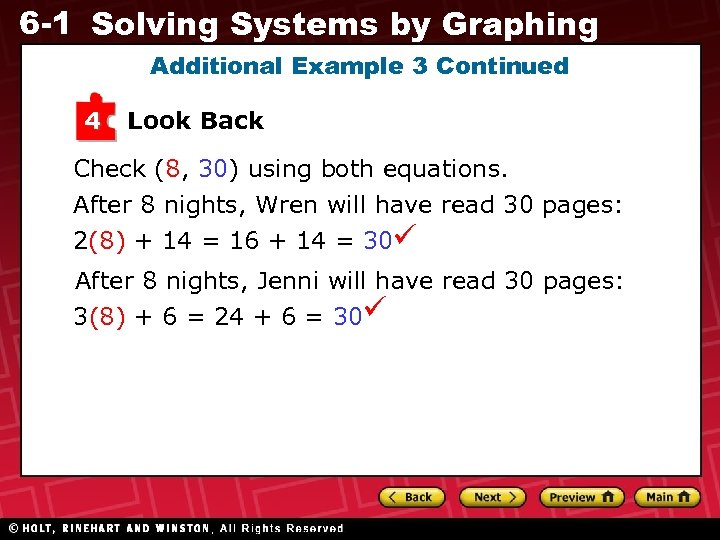6 -1 Solving Systems by Graphing Additional Example 3 Continued 4 Look Back Check (8, 30) using both equations. After 8 nights, Wren will have read 30 pages: 2(8) + 14 = 16 + 14 = 30 After 8 nights, Jenni will have read 30 pages: 3(8) + 6 = 24 + 6 = 306 -1 Solving Systems by Graphing Check It Out! Example 3 Video club A charges \$10 for membership and \$3 per movie rental. Video club B charges \$15 for membership and \$2 per movie rental. For how many movie rentals will the cost be the same at both video clubs? What is that cost?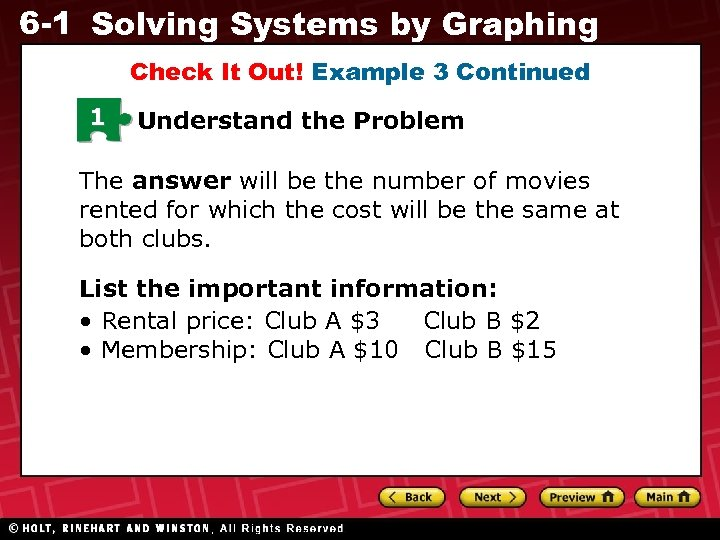6 -1 Solving Systems by Graphing Check It Out! Example 3 Continued 1 Understand the Problem The answer will be the number of movies rented for which the cost will be the same at both clubs. List the important information: • Rental price: Club A \$3 Club B \$2 • Membership: Club A \$10 Club B \$15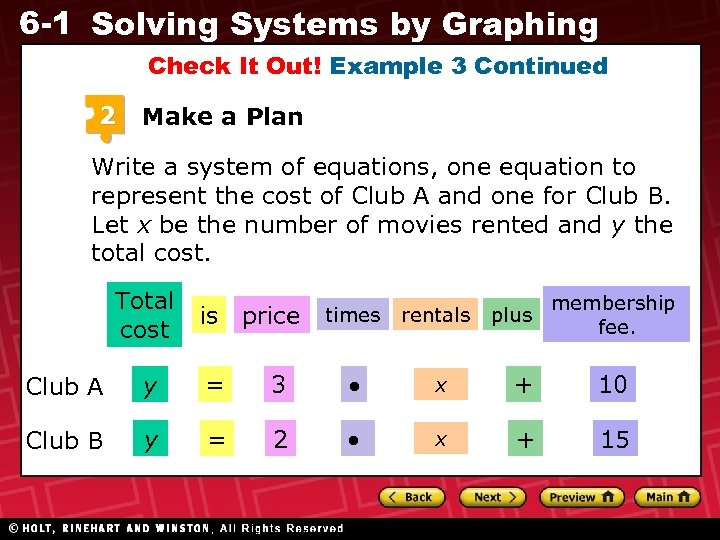6 -1 Solving Systems by Graphing Check It Out! Example 3 Continued 2 Make a Plan Write a system of equations, one equation to represent the cost of Club A and one for Club B. Let x be the number of movies rented and y the total cost. Total cost is price times rentals plus membership fee. Club A y = 3 x + 10 Club B y = 2 x + 15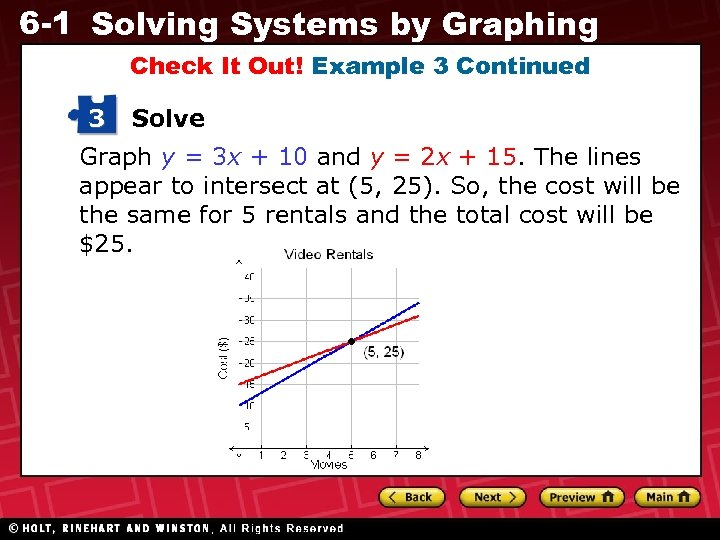6 -1 Solving Systems by Graphing Check It Out! Example 3 Continued 3 Solve Graph y = 3 x + 10 and y = 2 x + 15. The lines appear to intersect at (5, 25). So, the cost will be the same for 5 rentals and the total cost will be \$25.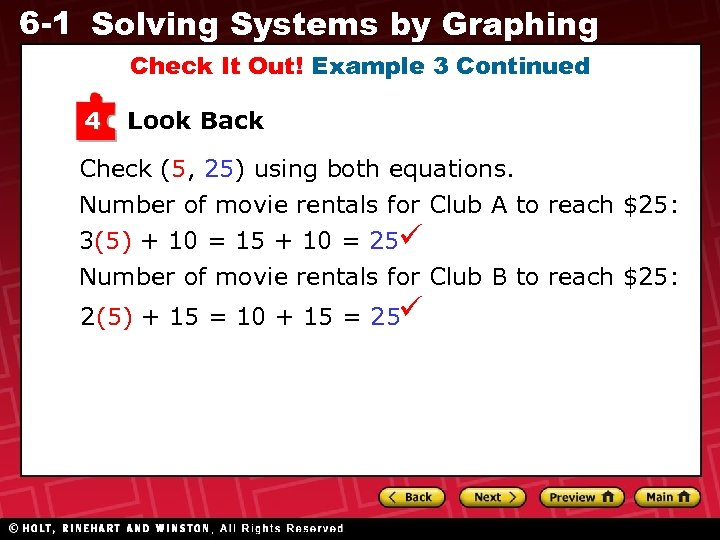6 -1 Solving Systems by Graphing Check It Out! Example 3 Continued 4 Look Back Check (5, 25) using both equations. Number of movie rentals for Club A to reach \$25: 3(5) + 10 = 15 + 10 = 25 Number of movie rentals for Club B to reach \$25: 2(5) + 15 = 10 + 15 = 25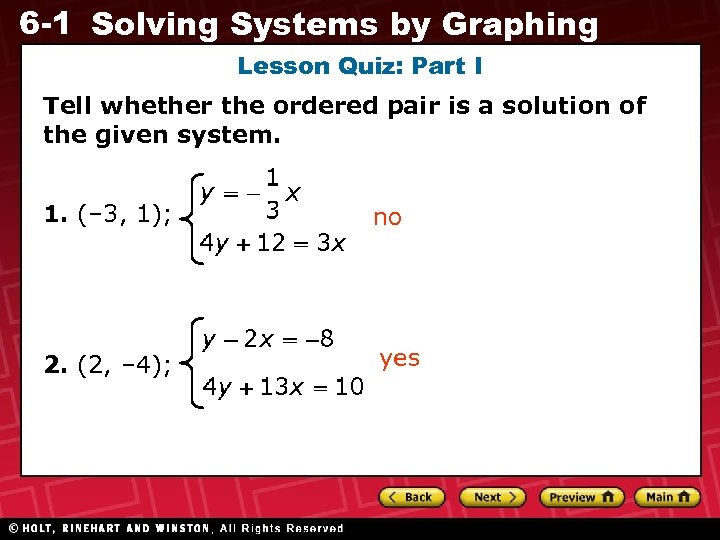6 -1 Solving Systems by Graphing Lesson Quiz: Part I Tell whether the ordered pair is a solution of the given system. 1. (– 3, 1); no 2. (2, – 4); yes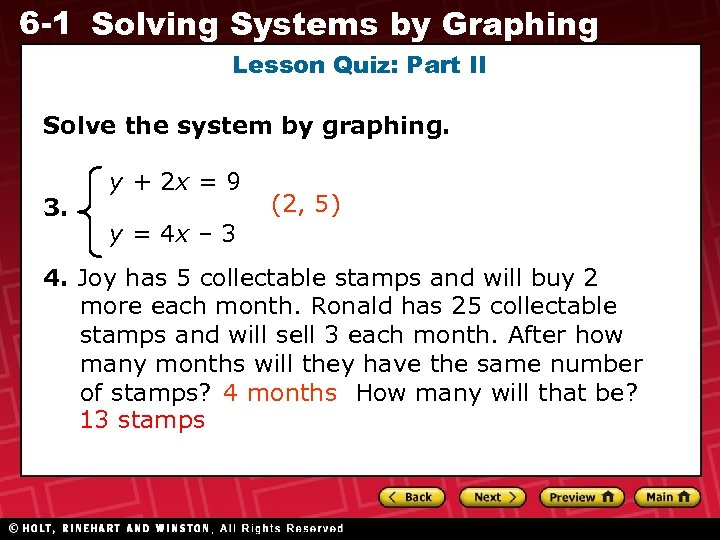6 -1 Solving Systems by Graphing Lesson Quiz: Part II Solve the system by graphing. 3. y + 2 x = 9 (2, 5) y = 4 x – 3 4. Joy has 5 collectable stamps and will buy 2 more each month. Ronald has 25 collectable stamps and will sell 3 each month. After how many months will they have the same number of stamps? 4 months How many will that be? 13 stamps